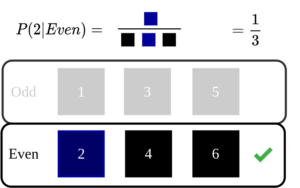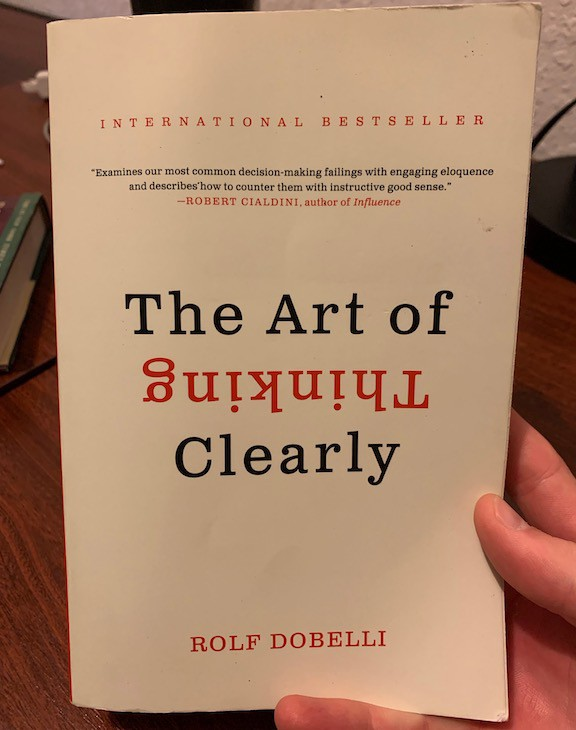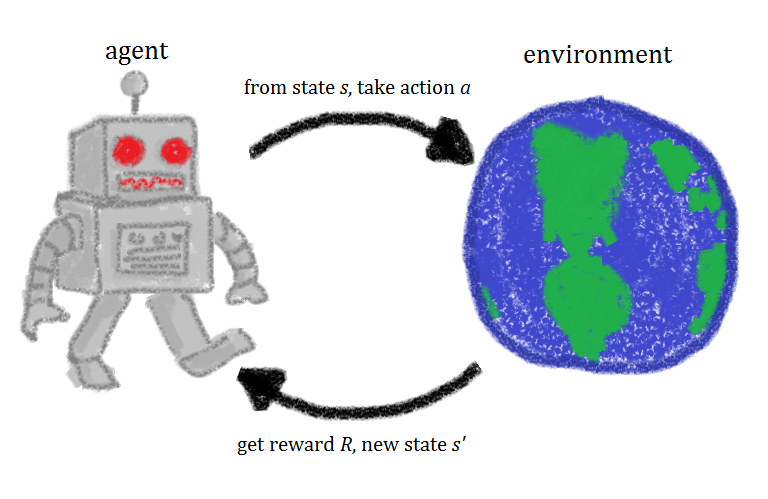# Month: December 2020## Reinforcement Learning Resources

Following is a list (in progress) of resources on Reinforcement Learning and allied topics. Though not comprehensive, it includes University lectures, YouTube playlists, MOOCs, blogs, etc. Hope this would be useful to someone getting started in reinforcement learning. UC Berkely CS 285 at UC Berkeley Deep Reinforcement Learning (23) Deep RL Bootcamp (15) CS 294: …## What does it mean to say orthogonal?

In this article, let us explore what is meant by the concept of orthogonality. Orthogonal or orthogonality is often introduced from a mathematical perspective. In general, most resources explains it in terms of linear algebra and vectors. One such example is when we learn about right-angled triangles the two sides are drawn as perpendicular or …## Mathematics for Machine Learning Resources

Every day, computational fields like machine learning, artificial intelligence, neuroscience, cryptography, etc. are making great progress. Anyone pursuing a career in all such fields might be looking for a solid foundation. If we dig deeper, we often trace back to the Queen of Science- Mathematics. How to learn mathematics for machine learning To learn mathematics …## An Intuitive Explanation of Naive Bayes Classifier

Introduction In this post, let’s take a look at the intuition behind Naive Bayes Classifier used in machine learning. Naive Bayes classifier is one of the basic algorithms often encountered in machine learning applications. If linear regression was based on concepts from linear algebra and calculus, naive Bayes classifier mostly backed up by probability theory. …

## Foundational Mathematics Courses

This article list out some of the foundational mathematics courses freely available on YouTube. A strong foundation flags off a voyage to knowledge and success. Anyone wish to have a great problem solving skill in science, technology or engineering will have to aquire the fundamental nuggets of mathematical ideas. This is because almost any problem …## Book summary: The Art Of Thinking Clearly by Rolf Dobelli

Hello, world, In this post, I summarize the book The Art Of Thinking Clearly by Rolf Dobelli. This book has some interesting observations about thinking clearly. I have listed out the main ideas in bold, its summary from respective chapters, along with my thoughts and view points. Introduction to Thinking Clearly Failure to think clearly …## Nine key papers in Distributional Reinforcement Learning Literature

In this post, I am going to give a summary of nine key papers from the distributional reinforcement learning (DRL) area. Paper 001 : A Distributional Perspective on Reinforcement Learning  This is the seminal paper in this area. The key idea of  the paper is the argument that the value distribution is important in reinforcement …## Paper Note: Distributional Reinforcement Learning with Quantile Regression

This article is a tutorial style discussion of the research paper titled “Distributional RL with Quantile Regression”. Here I try to reflect and convey the ideas of the paper with an intuitive style. Introduction Supervised machine learning deals with learning from examples. This is in contrast to real-life where we often don’t have a proper …